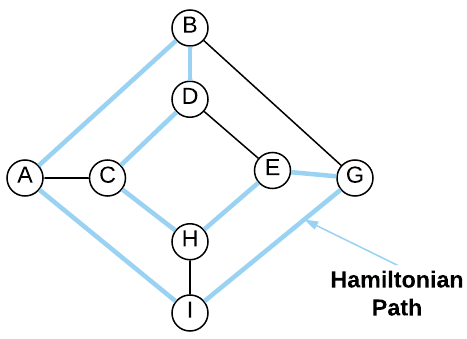# Traveling Salesman Problem

Under Construction: Consider this content a preview of the real thing which is coming soon (hopefully).

The Traveling Salesmen Problem (TSP) is without a doubt the most well-known riddle in computer science. It is in $NP$-hard; however, as discussed, this only means that an efficient algorithm for the general case has never been discovered. There are many tractable approaches.

The formulation of TSP is as follows: a salesperson must visit $n$ cities on a map; find the shortest (or cheapest) route that visits each city exactly once and ends in the same city in which it started. Formally stated, given a complete graph $G = (V, E)$ with non-negative integer costs $c(u, v)$ associated with each edge $(u, v) \in E$ find a Hamiltonian cycle (aka Hamiltonian tour) of $G$ with minimum cost.

A Hamiltonian cycle is a closed loop on an undirected graph where every vertex is visited exactly once. If a graph has more than one vertex and contains a Hamiltonian cycle, it’s known as a Hamiltonian graph. Every complete graph with more than two vertices is a Hamiltonian Graph. This is represented graphically below.As mentioned earlier, this is an $NP$-hard problem. Every complete graph has $(n-1)!/2$ Hamiltonian cycles. Assuming a single cycle can be evaluated in linear time ($O(n)$), a brute-force search through all possible cycles has a runtime of $\Theta(n!)$. It’s not possible (assuming $P \neq NP$) to find a polynomial time algorithm; however, it is possible to do better than a naive brute-force search. Listed below are a few approaches:

1. Dynamic Programming: The Bellman-Held-Karp algorithm is a dynamic programming algorithm that runs in $O(2^n n^2)$. Although not an ideal runtime, it enables far more use-cases than the brute-force version.
2. Approximation: There are several approximation algorithms that give near-optimal answers in polynomial time.
3. Cutting Planes (http://www.math.uwaterloo.ca/tsp/methods/dfj/index.html)

## Applications

Under Construction

## Asymptotic Complexity

Under Construction

## Pseudo Code

Under Construction

Full Repo

Relevant Files: